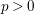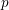Hostname: page-component-594f858ff7-7tp2g Total loading time: 0 Render date: 2023-06-07T03:15:28.132Z Has data issue: false Feature Flags: { "corePageComponentGetUserInfoFromSharedSession": false, "coreDisableEcommerce": false, "corePageComponentUseShareaholicInsteadOfAddThis": true, "coreDisableSocialShare": false, "useRatesEcommerce": true } hasContentIssue false

## Abstract

We show that the canonical lift construction for ordinary elliptic curves over perfect fields of characteristic$p>0$ extends uniquely to arbitrary families of ordinary elliptic curves, even over$p$-adic formal schemes. In particular, the universal ordinary elliptic curve has a canonical lift. The existence statement is largely a formal consequence of the universal property of Witt vectors applied to the moduli space of ordinary elliptic curves, at least with enough level structure. As an application, we show how this point of view allows for more formal proofs of recent results of Finotti and Erdoğan.

## MSC classification

Type
Article
Information
Nagoya Mathematical Journal , March 2019 , pp. 193 - 213
© 2017 Foundation Nagoya Mathematical Journal

## Access options

Get access to the full version of this content by using one of the access options below. (Log in options will check for institutional or personal access. Content may require purchase if you do not have access.)

## References

Artin, M., Grothendieck, A., Verdier, et J. L., Bourbaki, Avec la collaboration de N., Deligne, P. and Saint-Donat, et B., “ Séminaire de Géométrie Algébrique du Bois-Marie 1963–1964 (SGA 4) ”, in théorie des topos et cohomologie étale des schémas. Tome 1: Théorie des topos, Lecture Notes in Mathematics 269 , Springer, Berlin, 1972.Google Scholar
Artin, M., Grothendieck, A., Verdier, et J. L., Bourbaki, Avec la collaboration de N., Deligne, P. and Saint-Donat, et B., “ Séminaire de Géométrie Algébrique du Bois-Marie 1963–1964 (SGA 4) ”, in Théorie des topos et cohomologie étale des schémas. Tome 2, Lecture Notes in Mathematics 270 , Springer, Berlin, 1972.Google Scholar
Borger, J., The basic geometry of Witt vectors, I: the affine case , Algebra Number Theory 5(2) (2011), 231285.CrossRefGoogle Scholar
Borger, J., The basic geometry of Witt vectors. II: spaces , Math. Ann. 351(4) (2011), 877933.CrossRefGoogle Scholar
Buium, A., Differential characters of abelian varieties over p-adic fields , Invent. Math. 122(2) (1995), 309340.CrossRefGoogle Scholar
Buium, A., Differential modular forms , J. Reine Angew. Math. 520 (2000), 95167.Google Scholar
Erdoğan, A., A universal formula for the j-invariant of the canonical lifting , J. Number Theory 150 (2015), 2640.CrossRefGoogle Scholar
Finotti, L. R. A., Lifting the j-invariant: questions of Mazur and Tate , J. Number Theory 130(3) (2010), 620638.CrossRefGoogle Scholar
Gurney, L., Elliptic curves with complex multiplication and$\unicode[STIX]{x1D6EC}$ -structures, Ph.D. thesis, Australian National University, 2015, http://arxiv.org/abs/1710.08674.Google Scholar
Joyal, A., 𝛿-anneaux et vecteurs de Witt , C. R. Math. Rep. Acad. Sci. Can. 7(3) (1985), 177182.Google Scholar
Katz, N. M., “ p-adic properties of modular schemes and modular forms ”, in Modular Functions of One Variable, III (Proc. Internat. Summer School, Univ. Antwerp, Antwerp, 1972, Lecture Notes in Mathematics 350 , Springer, Berlin, 1973, 69190.Google Scholar
Katz, N. M. and Mazur, B., Arithmetic Moduli of Elliptic Curves, Annals of Mathematics Studies 108 , Princeton University Press, Princeton, NJ, 1985.CrossRefGoogle Scholar
Langer, A. and Zink, T., De Rham–Witt cohomology for a proper and smooth morphism , J. Inst. Math. Jussieu 3(2) (2004), 231314.CrossRefGoogle Scholar
Lazard, M., Commutative Formal Groups, Lecture Notes in Mathematics 443 , Springer, Berlin–New York, 1975.CrossRefGoogle Scholar
Messing, W., The Crystals Associated to Barsotti–Tate Groups: with Applications to Abelian Schemes, Lecture Notes in Mathematics 264 , Springer, Berlin–New York, 1972.CrossRefGoogle Scholar
The Stacks Project Authors, Stacks Project. http://stacks.math.columbia.edu, 2016.Google Scholar
van der Kallen, W., Descent for the K-theory of polynomial rings , Math. Z. 191(3) (1986), 405415.CrossRefGoogle Scholar